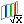# Element Forces (Tensor Plot)

2D element forces and moments are supported as tensors and can be plotted using the Tensor panel in HyperView.

This functionality is only available for the .op2 file. Element force and moment data is requested using the ELFORCE or FORCE case control command.

## Element X-Axis

In order to visualize element forces and moments with a proper sense of orientation, the element x-axis must first be identified. Refer to the CQUAD4/CTRIA3 documentation in the Radioss, MotionSolve, and OptiStruct Guide for more information on how to define the element x-axis for 2D elements.

Load the model and results files with the Advanced Result-Math template in order to visualize the element x-axis in HyperView.

Once the results are loaded, the Derived Results iconwill launch the Expression Builder allowing you to define model or results-specific datatypes.

The Expression Builder is a graphical user interface which allows user-defined data type expressions to be authored directly within HyperView. These expressions use a simplified Templex-style syntax that gets parsed into XML statements which are then passed on to Result Math for processing. Refer to the HyperView Expression Builder documentation for more information.

The element x-axis vector can be added to the list of datatypes by double clicking ElementAxisVector from the Model Operator. Use a meaningful label so this expression can be identified amongst the other vector datatypes while plotting.

Below is a vector plot of each 2D element x-axis.
When the element x-axis vector plot is overlaid on a force or moment tensor plot, the positive and negative X, Y and XY values become clear.

## Forces

The image below shows the positive directions for element forces in a 2D element.
• Fx and Fy are the normal forces acting on the x and y faces of the 2D element below and are represented by the XX and YY components in the Regular drawing mode of the tensor plot in HyperView.
• Fxy is the in-plane shear force and is represented by the XY component in the Regular drawing mode of the tensor plot in HyperView.
• Vx and Vy are the transverse shear forces acting on the x and y faces of the 2D element below and are represented by the ZX and YZ components in the Regular drawing mode of the tensor plot in HyperView.

## Moments

The image below shows the positive directions for element moments in a 2D element.
• Mx and My are the bending moments acting on the x and y faces of the 2D element below and are represented by the XX and YY components in the Moment drawing mode of the tensor plot in HyperView.
• Mxy is the twisting moment and is represented by the XY component in the Moment drawing mode of the tensor plot in HyperView.

## Plotting Element Forces and Moments using the Tensor Panel

There are a number of options in the tensor panel and since it is used to plot more than just element forces and moments, some of the options are not meant to be used while plotting forces and moments.
Resolved in
Element forces and moments are always written to the .op2 file in the elemental system. If there is a material system defined on the 2D elements, and PARAM, OMID is used, these forces and moments can be transformed and written to that system, but only in an ASCII output.
Now that element forces and moments are read into HyperView as tensors they can be transformed from the elemental system to any other system, even a system created on the fly in HyperView.
Tensor Format
The Tensor format should be set to Component. This allows any or all of the full tensor components to be plotted. The Principal Tensor format is used for plotting stress tensors.
Display Options
Size scaling and color by options are rather straightforward. For clear results normalize the tensor to a value of 1.0 or less. This will ensure that the tensor drawings will not breech the elements’ edge.
There are two options available for the Draw mode: Regular and Moment.
Draw mode: Regular
Regular drawing mode will plot a traditional full tensor for each element. This is typically used for stress and element force tensor plots, and useful to properly visualize tensor transformations.
Draw mode: Moment
The Moment drawing mode was specially added to better conceptualize 2D element moments. This mode is not useful for transformations, and only applies for element moments, but it will allow you to better understand the physics behind the subcase without needing an element reference guide. The Regular drawing mode can be used to visualize any tensor, including element moments, but the Moment drawing mode was specifically designed for plotting element moments themselves.